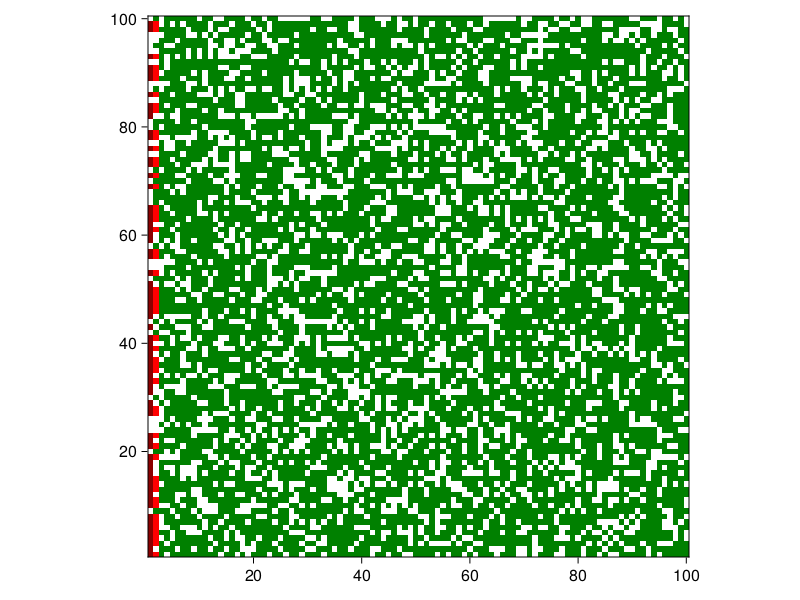# Forest fire

The forest fire model is defined as a cellular automaton on a grid. A position can be empty or occupied by a tree which is ok, burning or burnt. We implement a slightly different ruleset to that of Drossel and Schwabl (1992), so that our implementation can be compared with other ABM frameworks

1. A burning position turns into a burnt position
2. A tree will burn if at least one neighbor is burning

The forest has an innate density, which is the proportion of trees initialized as green, however all trees that reside on the left side of the grid are burning. The model is also available from the Models module as Models.forest_fire.

## Defining the core structures

Cellular automata don't necessarily require an agent-like structure. Here we will demonstrate how a model focused solution is possible.

using Agents, Random
using InteractiveDynamics
using CairoMakie

@agent Automata GridAgent{2} begin end

The agent type Automata is effectively a dummy agent, for which we will invoke dummystep when stepping the model.

We then make a setup function that initializes the model.

function forest_fire(; density = 0.7, griddims = (100, 100), seed = 2)
space = GridSpaceSingle(griddims; periodic = false, metric = :manhattan)
rng = Random.MersenneTwister(seed)
# The trees field is coded such that
# Empty = 0, Green = 1, Burning = 2, Burnt = 3
forest = ABM(Automata, space; rng, properties = (trees = zeros(Int, griddims),))
for I in CartesianIndices(forest.trees)
if rand(forest.rng) < density
# Set the trees at the left edge on fire
forest.trees[I] = I == 1 ? 2 : 1
end
end
return forest
end

forest = forest_fire()
AgentBasedModel with 0 agents of type Automata
space: GridSpaceSingle with size (100, 100), metric=manhattan, periodic=false
scheduler: fastest
properties: trees

## Defining the step!

function tree_step!(forest)
# Find trees that are burning (coded as 2)
for I in findall(isequal(2), forest.trees)
for idx in nearby_positions(I.I, forest)
# If a neighbor is Green (1), set it on fire (2)
if forest.trees[idx...] == 1
forest.trees[idx...] = 2
end
end
# Finally, any burning tree is burnt out (2)
forest.trees[I] = 3
end
end
tree_step! (generic function with 1 method)

## Running the model

Agents.step!(forest, dummystep, tree_step!, 1)
count(t == 3 for t in forest.trees) # Number of burnt trees on step 1
70
Agents.step!(forest, dummystep, tree_step!, 10)
count(t == 3 for t in forest.trees) # Number of burnt trees on step 11
560

Now we can do some data collection as well using an aggregate function percentage:

forest = forest_fire(griddims = (20, 20))
burnt_percentage(f) = count(t == 3 for t in f.trees) / prod(size(f.trees))
mdata = [burnt_percentage]

_, data = run!(forest, dummystep, tree_step!, 10; mdata)
data
11×2 DataFrame
Rowstepburnt_percentage
Int64Float64
100.0
210.0375
320.065
430.0925
540.1125
650.135
760.16
870.1925
980.215
1090.245
11100.27

Now let's plot the model. We use green for unburnt trees, red for burning and a dark red for burnt.

forest = forest_fire()
Agents.step!(forest, dummystep, tree_step!, 1)

plotkwargs = (
heatarray = :trees,
heatkwargs = (
colorrange = (0, 3),
colormap = cgrad([:white, :green, :red, :darkred]; categorical = true),
),
)
fig, _ = abmplot(forest; plotkwargs...)
figor animate it

forest = forest_fire(density = 0.7, seed = 10)
abmvideo(
"forest.mp4",
forest,
dummystep,
tree_step!;
as = 0,
framerate = 5,
frames = 20,
spf = 5,
title = "Forest Fire",
plotkwargs...,
)
┌ Warning: Unknown agent position type: Vector{Any}. Skipping plotting agents.
└ @ InteractiveDynamics ~/.julia/packages/InteractiveDynamics/EThtU/src/agents/abmplot.jl:253